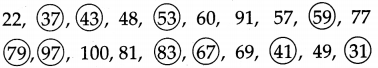# Maharashtra Board Class 5 Maths Solutions Chapter 8 Multiples and Factors Problem Set 34

Balbharti Maharashtra Board Class 5 Maths Solutions Chapter 8 Multiples and Factors Problem Set 34 Textbook Exercise Important Questions and Answers.

## Maharashtra State Board Class 5 Maths Solutions Chapter 8 Multiples and Factors Problem Set 34

Question 1.
Write all the prime numbers between 1 and 20.
2, 3, 5, 7, 11, 13, 17, 19.

Question 2.
Write all the composite numbers between 21 and 50.
Composite numbers between 21 and 50 are 22, 24, 25, 26, 27, 28, 30, 32, 33, 34, 35, 36, 38, 39, 40, 42, 44, 45, 46, 48, 49.

Question 3.
Circle the prime numbers in the list given below. 22, 37, 43, 48, 53, 60, 91, 57, 59, 77, 79, 97, 100Question 4.
Which of the prime numbers are even numbers?
Only even prime number is 2. (the Rest of the even numbers are composites.)

Co-prime numbers

Dada : Tell me all the factors of 12 and 18.

Anju : I’ll tell the factors of 12: 1, 2, 3, 4, 6, 12.

Manju : I’ll give the factors of 18: 1, 2, 3, 6, 9, 18.

Dada : Now find the common factors of 12 and 18.

Anju : Common factors ?

Dada : 1, 2, 3 and 6 are in both groups, which means that they are common factors. Now tell me the factors of 10 and 21.

Sanju : Factors of 10 : 1, 2, 5, 10.

Manju : Factors of 21: 1, 3, 7, 21.

Dada : Which of the factors in these two groups are common?

Sanju : 1 is the only common factor.

Dada : Numbers which have only 1 as a common factor are called co-prime numbers, so 10 and 21 are co-prime numbers. The common factors of 12 and 18 are 1, 2, 3 and 6; which means that the common factors are more than one. Therefore, 12 and 18 are not co-prime numbers. Now tell me whether 8 and 10 are co-prime numbers.

Manju : The factors of 8 are 1, 2, 4 and 8 and the factors of 10 are 1, 2, 5 and 10. These numbers have two factors, 1 and 2, in common, so 8 and 10 are not co-prime numbers.

Question 1.
21 to 50
23, 29, 31, 37, 41, 43, 47

Question 2.
Which of the number is neither prime nor composite?